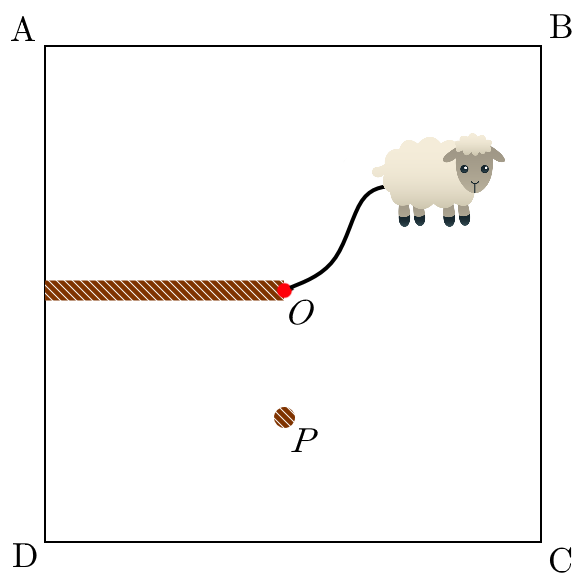# Larry The Lamb

Geometry Level 2A square field $ABCD$ has center $O$ and side length $2l$. A rope of length $l$ connects Larry the lamb at one end to $O$ at the other end.

The perpendicular from $O$ to side $AD$ forms a wall that Larry cannot pass through. The point $P$ that is the midpoint of the perpendicular from $O$ to $DC$ forms a peg that will catch on the rope.

What percentage of the field can Larry walk on, to the nearest percent?

×

Problem Loading...

Note Loading...

Set Loading...